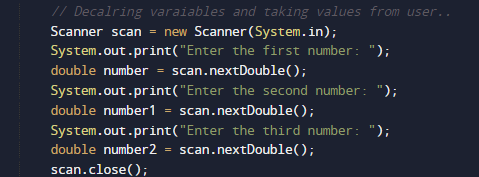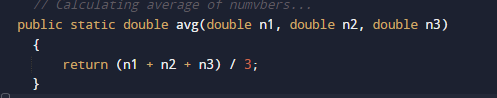# Java Program to Find average of 3 numbers

In this tutorial you will learn about the Java Program to Find average of 3 numbers and its application with practical example.

In this tutorial, we will learn to create a Java Program to Find average of 3 numbers using Java programming.

## Prerequisites

Before starting with this tutorial we assume that you are best aware of the following Java programming topics:

• Java Operators.
• Basic Input and Output function in Java.
• Class and Object in Java.
• Basic Java programming.
• If-else statements in Java.
• For loop in Java.

## What is average of numbers.

Average of numbers is by adding up all the numbers and dividing by size of numbers are in the set.

## Java Program to Find average of 3 numbers

In this program we would find average of given number.first of we would take a values from user and find the average of  numbers.let have a look at the code.

## Output

Average of Three Numbers.In the above program, we have first declared and initialized a set variables required in the program.

• number,number1 and number2 = it will hold entered numbers.

After that we take a numbers from user and find average of numbers.after getting values from users we pass the number to function avg().In this Function we take three parameter as a arguments and add all three values and divide the sum by 3 to get the desire output and return the average to main function and where we will print the result of program.Above image is the output of above program.

In this tutorial we have learn about the Java Program to Find average of 3 numbers and its application with practical example. I hope you will like this tutorial.